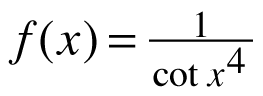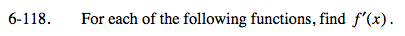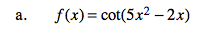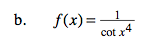### Home > CALC > Chapter 6 > Lesson 6.3.4 > Problem6-118

6-118.
1. For each of the following functions, find f ′(x). Homework Help ✎

1. f(x) = cot(5x2 − 2x)

2.$\text{Recall that }\frac{d}{dx}\cot(x)=-\csc^{2}(x).$Before differentiating, rewrite the expression.

Note that cot(x4) ≠ cot4(x).